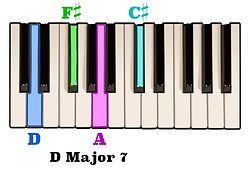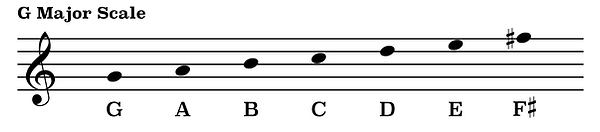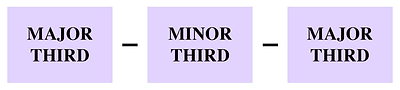top of page

## Seventh Chord Construction | Major Seventh Chords (1 of 5)

The notes of a Major seventh chord consist of the notes of a Major triad with the addition of an interval of a Major seventh above the root note.For example, the notes of a C Major triad are C, E, and G,and an interval of a Major seventh above the note C is the note B.Therefore, by adding the note B to the notes of a C Major triad we will have created a C Major seventh chord.Likewise, the notes of a D Major triad are D, F-sharp, and A.If we add an interval of a Major seventh above the root note D to the notes of a D Major triadwe will have created a D Major seventh chord.

Adding an interval of a Major seventh above the root note of any Major triad will always result in a Major seventh chord.

Now, because the notes of a Major triad come from the first, third, and fifth scale degrees of a Major scale, and an interval of a Major seventh above any given note can always be found as the seventh scale degree of that note's Major scale, another way to think about how to construct a Major seventh chord is by simply combining the first, third, fifth, and seventh scale degrees of a corresponding Major scale.

Here are the notes of a G Major scale.We can see that the first scale degree is the note G, the third scale degree is the note B, the fifth scale degree is the note D, and the seventh scale degree is the note F-sharp.By playing these four notes, either melodically or harmonically, we will have created a G Major seventh chord.It can also be helpful to think about Major seventh chords in terms of the intervals which separate each consecutive note. For example, the interval pattern which produces a Major seventh chord is Major third, minor third, Major third.In other words, to construct an F Major seventh chord first play an interval of a Major third above the note F, followed by an interval of a minor third above the note A, followed by an interval of a Major third above the note C.By following this pattern of intervals you will be able to create a Major seventh chord above any root note.

bottom of page